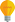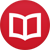# Integral of $\cos^2(x)$

by Jakub MarianTip: See my list of theMost Common Mistakes in English. It will teach you how to avoid mis­takes with com­mas, pre­pos­i­tions, ir­reg­u­lar verbs, and much more.

The easiest way to calculate this integral is to use a simple trick. First, we write $\cos^2(x) = \cos(x)\cos(x)$ and apply integration by parts:

$$∫ \cos(x)\cos(x)\,dx = \cos(x)\sin(x)-∫(-\sin(x))(\sin(x))\,dx$$

If we apply integration by parts to the rightmost expression again, we will get $∫\cos^2(x)dx = ∫\cos^2(x)dx$, which is not very useful. The trick is to rewrite the $\sin^2(x)$ in the second step as $1-\cos^2(x)$. Then we get

\begin{align*} ∫ \cos^2(x)\,dx &= \cos(x)\sin(x)+∫(1-\cos^2(x))\,dx \\ &= \cos(x)\sin(x)+x-∫\cos^2(x)\,dx \end{align*}

Now, all we have to do is to get $∫\cos^2(x)\,dx$ from the right-hand side to the left-hand side of the equation:

$$2∫ \cos^2(x)\,dx = \cos(x)\sin(x)+x$$ $$∫ \cos^2(x)\,dx = ÷{1}{2}(\cos(x)\sin(x)+x)+c$$

One last question remains: where the hell did the $+c$ come from? I will answer that in a separate article in the future.

By the way, I have written several educational ebooks. If you get a copy, you can learn new things and support this website at the same time—why don’t you check them out?